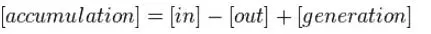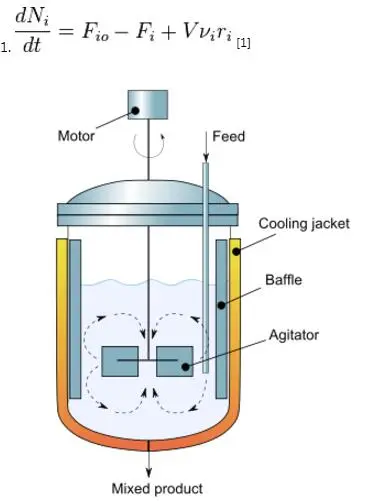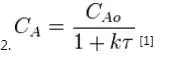Continuous stirred-tank reactor

# Continuous stirred-tank reactor

The continuous flow stirred-tank reactor (CSTR), also known as vat- or backmix reactor, is a common ideal reactor type in chemical engineering. A CSTR often refers to a model used to estimate the key unit operation variables when using a continuous[†] agitated-tank reactor to reach a specified output. (See Chemical reactors.) The mathematical model works for all fluids: liquids, gases, and slurries.

The behavior of a CSTR is often approximated or modeled by that of a Continuous Ideally Stirred-Tank Reactor (CISTR). All calculations performed with CISTRs assume perfect mixing. In a perfectly mixed reactor, the output composition is identical to composition of the material inside the reactor, which is a function of residence time and rate of reaction. If the residence time is 5-10 times the mixing time, this approximation is valid for engineering purposes. The CISTR model is often used to simplify engineering calculations and can be used to describe research reactors. In practice it can only be approached, in particular in industrial size reactors.

Assume:

• perfect or ideal mixing, as stated above

Integral mass balance on number of moles Ni of species i in a reactor of volume V.Cross-sectional diagram of Continuous flow stirred-tank reactor

where Fio is the molar flow rate inlet of species iFi the molar flow rate outlet, and$\nu_i$ stoichiometric coefficient. The reaction rate, r, is generally dependent on the reactant concentration and the rate constant (k). The rate constant can be determined by using a known empirical reaction rates that is adjusted for temperature using the Arrhenius temperature dependence. Generally, as the temperature increases so does the rate at which the reaction occurs. Residence time,$\tau$, is the average amount of time a discrete quantity of reagent spends inside the tank.

Assume:

• constant density (valid for most liquids; valid for gases only if there is no net change in the number of moles or drastic temperature change)

• isothermal conditions, or constant temperature (k is constant)

• single, irreversible reaction (νA = -1)

• first-order reaction (r = kCA)

A → products

NA = CA V (where CA is the concentration of species A, V is the volume of the reactor, NA is the number of moles of species A)The values of the variables, outlet concentration and residence time, in Equation 2 are major design criteria.

To model systems that do not obey the assumptions of constant temperature and a single reaction, additional dependent variables must be considered. If the system is considered to be in unsteady-state, a differential equation or a system of coupled differential equations must be solved.

CSTR's are known to be one of the systems which exhibit complex behavior such as steady-state multiplicity, limit cycles and chaos.

 产品中心 解决方案 乳化机反应釜分散机研磨机搅拌机灌装机不锈钢储罐水处理设备原浆发酵设备自动生产系统 化妆品生产设备膏霜生产设备液洗生产设备香水生产设备口红生产设备雾泡罐生产设备粉饼生产设备牙膏生产设备香皂生产设备化工原料与助剂生产设备醇酸树脂生产设备丙烯酸树脂生产设备 （PU）聚氨酯环氧树脂生产设备多元醇生产设备硅油、光油生产设备热熔胶生产设备改性松香树脂生产设备粘合剂生产设备减水剂生产设备LED原料搅拌生产设备水性丙烯酸生产设备水性PU树脂生产设备涂料生产设备 油墨生产设备新能源材料生产设备润滑油生产设备车用燃料生产设备食品添加剂生产设备制药生产设备软膏生产设备糖浆生产设备针剂药水生产设备互联网应用

86-20-39900130

• 官方微信
• 官方微博